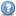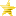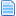# Lifebars in Character (Read 7176 times)

Started by BlackFired, September 20, 2015, 06:31:20 pm
Share this topic:

#### BlackFired##### Lifebars in Character
#1  September 20, 2015, 06:31:20 pm
••• M.U.G.E.N. Developer
• Hello Baby :\$
Hi, this is my code:
In the Statedef -2.

Explod of the Lifes`bar:
Code:
`;Lifebar[State 0, Explod]type = Explodtrigger1 = var(32) = 10anim = 3000ID = 3000pos = 145,12space = screen;postype = front  ;p2,front,back,left,rightfacing = 1vfacing = 1bindtime = 1pausemovetime = 0scale = 0.3,0.3sprpriority = -2ignorehitpause = 1[State 0, Explod]type = Explodtrigger1 = var(32) = 10anim = 3001ID = 3001pos = 145,12space = screen;postype = front;,back,left,rightfacing = 1vfacing = 1bindtime = 1pausemovetime = 0scale = 0.3,0.3sprpriority = -2ignorehitpause = 1[State 0, Explod]type = Explodtriggerall = var(32) = 10trigger1   = life = lifemaxanim = 3002ID = 3002pos = 143,12space = screen;postype = front;,back,left,rightfacing = 1vfacing = 1bindtime = 1pausemovetime = 0scale = 0.3,0.3;0.3sprpriority = 0ignorehitpause = 1`

Explod of the Power`bar
Code:
`;PowerBar[State 0, Explod]type = Explodtrigger1 = var(32) = 10anim = 3003ID = 3003pos = 143,27;35,-165space = screen;postype = front ;,back,left,rightfacing = 1vfacing = 1bindtime = 1pausemovetime = 0scale = 0.3,0.3sprpriority = -2ignorehitpause = 1[State 0, Explod]type = Explodtrigger1 = var(32) = 10anim = 3004ID = 3004pos = 143,27;35,-165space = screen;postype = front  ;p2,front,back,left,rightfacing = 1vfacing = 1bindtime = 1pausemovetime = 0scale = 0.3,0.3sprpriority = -2ignorehitpause = 1[State 0, Explod]type = Explodtriggerall = var(32) = 10trigger1 = power = powermaxanim = 3005ID = 3005pos = 143,27;35,-165space = screen;postype = front  ;p2,front,back,left,rightfacing = 1vfacing = 1bindtime = 1pausemovetime = 0scale = 0.3,0.3sprpriority = 0ignorehitpause = 1`

To control the duration of explod
Code:
`;Vars Para Controlar Tiempo[State 0, VarSet]type = VarSettrigger1 = 1fv = 32 value = 10ignorehitpause = 1[State 0, VarSet]type = VarSettrigger1 = 1v = 32value = 10ignorehitpause = 1;Tiempo en Control {Fv(32)=10}[State 0, ExplodBindTime]type = ExplodBindTimetrigger1 =  1ID = 3000time = fvar(32) = 10ignorehitpause = 1[State 0, ExplodBindTime]type = ExplodBindTimetrigger1 =  1ID = 3001time = fvar(32) = 10ignorehitpause = 1[State 0, ExplodBindTime]type = ExplodBindTimetrigger1 =  1ID = 3002time = fvar(32) = 10ignorehitpause = 1[State 0, ExplodBindTime]type = ExplodBindTimetrigger1 =  1ID = 3003time = fvar(32) = 10ignorehitpause = 1[State 0, ExplodBindTime]type = ExplodBindTimetrigger1 =  1ID = 3004time = fvar(32) = 10ignorehitpause = 1[State 0, ExplodBindTime]type = ExplodBindTimetrigger1 =  1ID = 3005time = fvar(32) = 10ignorehitpause = 1`

Now the dilemma, I need a formula that reduces the bars, according to the percentage of life and energy. He probado con lo siguiente:
Code:
`scale = 0+life,0.3`

Unfortunately, that did not work, either causes error, if any help possible. Thx

#### ALD##### Re: Lifebars in Character
#2  September 23, 2015, 03:00:32 am
••I have not really checked how it works but if all you want is to be scaled according to the life:

[State -2]
type = ModifyExplod
trigger1 = NumExplod(\$) = \$ && TeamSide = \$
scale = 1/LifeMax*Life,1
id = \$
facing = \$
pausemovetime = \$
supermovetime = \$